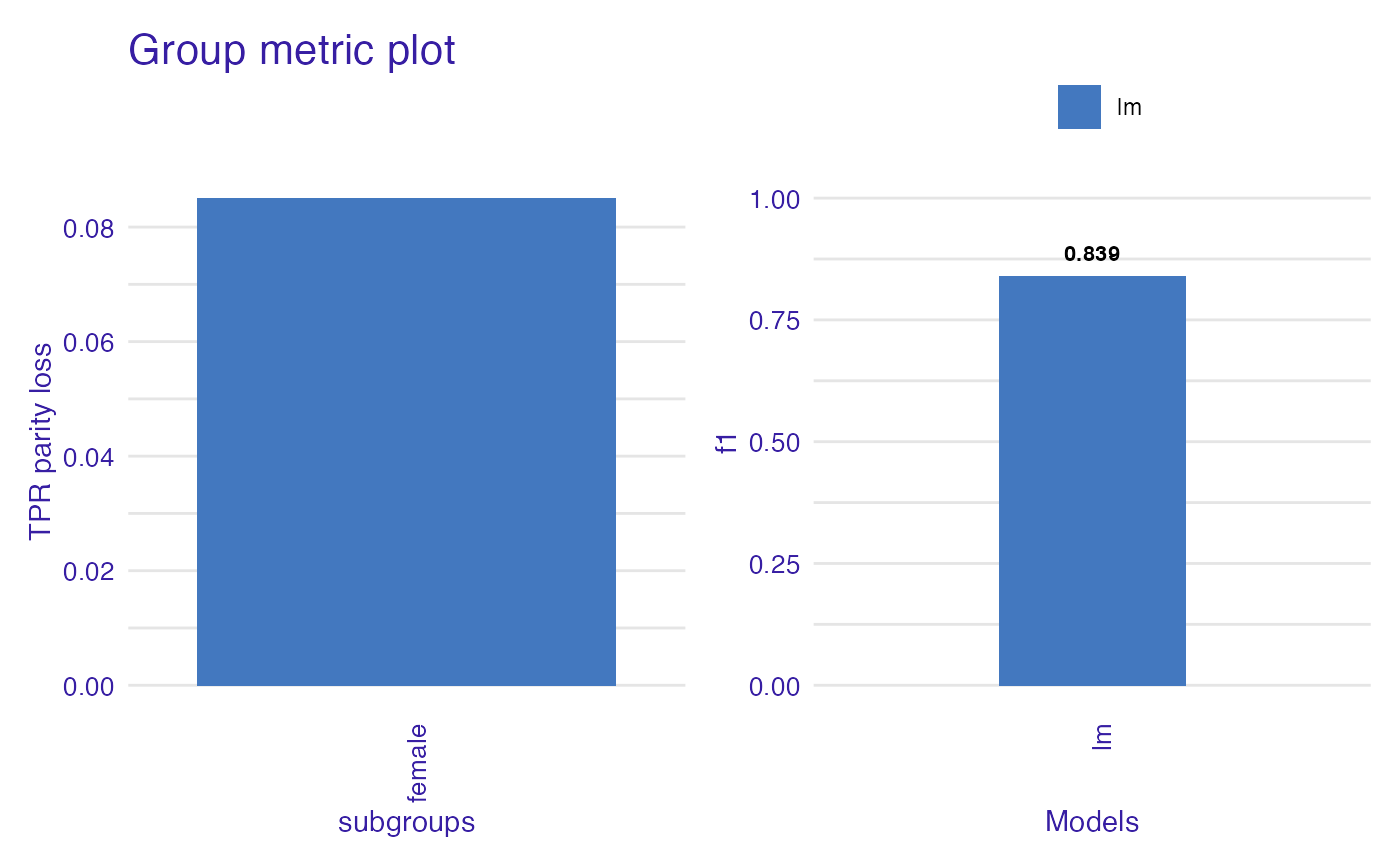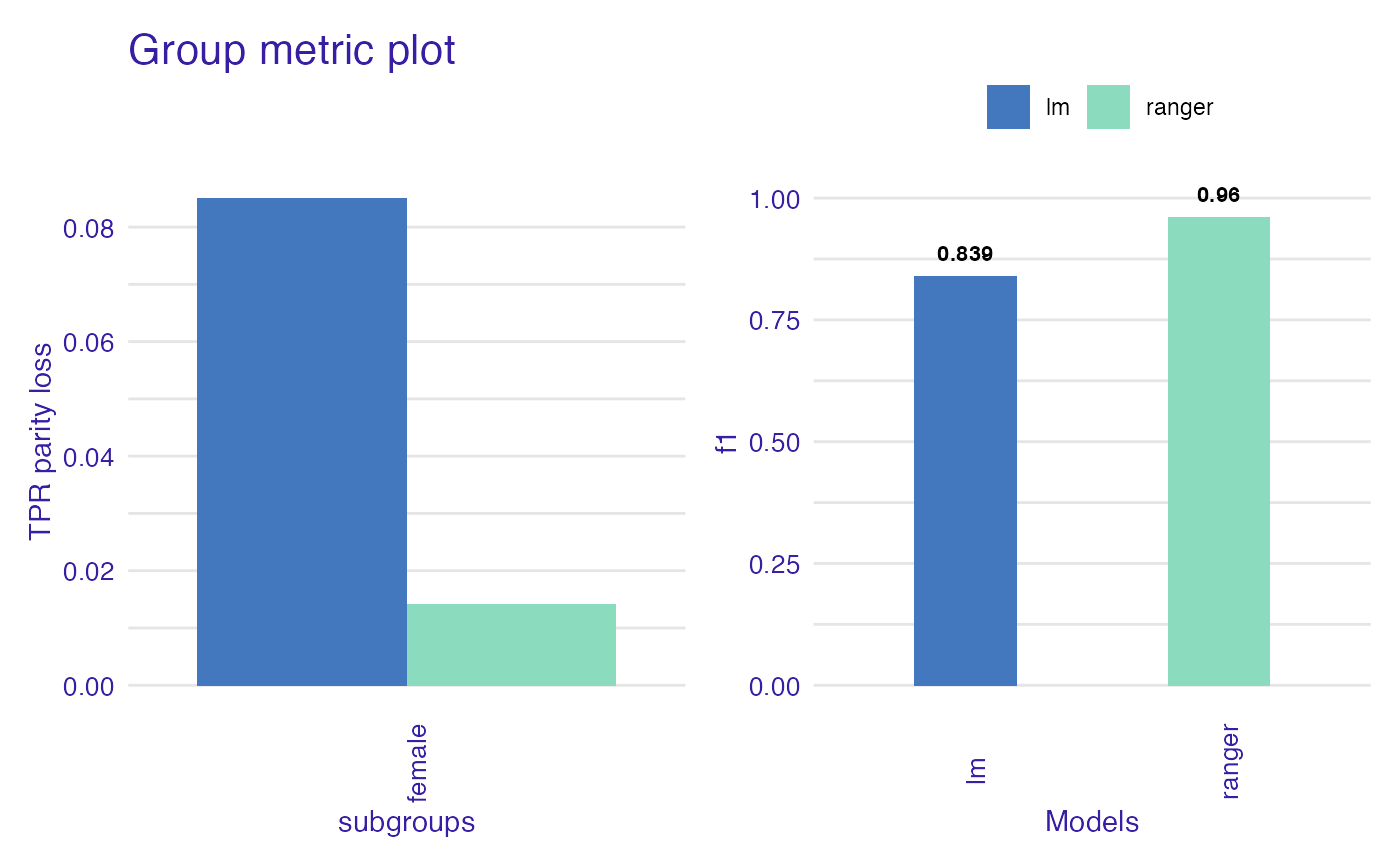Group metric enables to extract data from metrics generated for each subgroup (values in protected variable) The closer metric values are to each other, the less bias particular model has. If parity_loss parameter is set to TRUE, distance between privileged and unprivileged subgroups will be measured. When plotted shows both fairness metric and chosen performance metric.

group_metric(
x,
fairness_metric = NULL,
performance_metric = NULL,
parity_loss = FALSE,
verbose = TRUE
)

Arguments

x object of class fairness_object character, fairness metric name, if NULL the default metric will be used which is TPR. character, performance metric name logical, if TRUE parity loss will supersede basic metric logical, whether to print information about metrics on console or not. Default TRUE

Value

group_metric object. It is a list with following items:

• group_metric_data - data.frame containing fairness metric scores for each model

• performance_data - data.frame containing performance metric scores for each model

• fairness_metric - name of fairness metric

• performance_metric - name of performance metric

Details

Available metrics:

Fairness metrics (Full names explained in fairness_check documentation):

• TPR

• TNR

• PPV

• NPV

• FNR

• FPR

• FDR

• FOR

• TS

• ACC

• STP

• F1

Performance metrics

• recall

• precision

• accuracy

• f1

• auc

Examples

data("german")

y_numeric <- as.numeric(german$Risk) - 1 lm_model <- glm(Risk ~ ., data = german, family = binomial(link = "logit") ) explainer_lm <- DALEX::explain(lm_model, data = german[, -1], y = y_numeric) #> Preparation of a new explainer is initiated #> -> model label : lm ( default ) #> -> data : 1000 rows 9 cols #> -> target variable : 1000 values #> -> predict function : yhat.glm will be used ( default ) #> -> predicted values : No value for predict function target column. ( default ) #> -> model_info : package stats , ver. 4.1.1 , task classification ( default ) #> -> predicted values : numerical, min = 0.1369187 , mean = 0.7 , max = 0.9832426 #> -> residual function : difference between y and yhat ( default ) #> -> residuals : numerical, min = -0.9572803 , mean = 1.940006e-17 , max = 0.8283475 #> A new explainer has been created! fobject <- fairness_check(explainer_lm, protected = german$Sex,
privileged = "male"
)
#> Creating fairness classification object
#> -> Privileged subgroup		: character ( Ok  )
#> -> Protected variable		: factor ( Ok  )
#> -> Cutoff values for explainers	: 0.5 ( for all subgroups )
#> -> Fairness objects		: 0 objects
#> -> Checking explainers		: 1 in total (  compatible  )
#> -> Metric calculation		: 13/13 metrics calculated for all models
#>  Fairness object created succesfully

gm <- group_metric(fobject, "TPR", "f1", parity_loss = TRUE)
#>
#> Creating object with:
#> Fairness metric:  TPR
#> Performance metric:  f1
#>
plot(gm)# \donttest{

rf_model <- ranger::ranger(Risk ~ .,
data = german,
probability = TRUE,
num.trees = 200
)

explainer_rf <- DALEX::explain(rf_model, data = german[, -1], y = y_numeric)
#> Preparation of a new explainer is initiated
#>   -> model label       :  ranger  (  default  )
#>   -> data              :  1000  rows  9  cols
#>   -> target variable   :  1000  values
#>   -> predict function  :  yhat.ranger  will be used (  default  )
#>   -> predicted values  :  No value for predict function target column. (  default  )
#>   -> model_info        :  package ranger , ver. 0.13.1 , task classification (  default  )
#>   -> predicted values  :  numerical, min =  0.07845238 , mean =  0.6954966 , max =  0.9985714
#>   -> residual function :  difference between y and yhat (  default  )
#>   -> residuals         :  numerical, min =  -0.7493194 , mean =  0.004503438 , max =  0.6740554
#>   A new explainer has been created!

fobject <- fairness_check(explainer_rf, fobject)
#> Creating fairness classification object
#> -> Privileged subgroup		: character ( from first fairness object  )
#> -> Protected variable		: factor ( from first fairness object  )
#> -> Cutoff values for explainers	: 0.5 ( for all subgroups )
#> -> Fairness objects		: 1 object (  compatible  )
#> -> Checking explainers		: 2 in total (  compatible  )
#> -> Metric calculation		: 10/13 metrics calculated for all models ( 3 NA created )
#>  Fairness object created succesfully

gm <- group_metric(fobject, "TPR", "f1", parity_loss = TRUE)
#>
#> Creating object with:
#> Fairness metric:  TPR
#> Performance metric:  f1
#>

plot(gm)# }